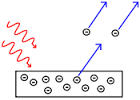# Photoelectric Effect

The photoelectric effect is the observation that many metals emit electrons when light shines upon them. Electrons emitted in this manner can be called photoelectrons.

The work function of an element is the minimum energy needed to remove an electron from the solid element. The work function for rubidium is $\SI[per-mode=symbol]{208.4}{\kilo\joule\per\mole}$.

What is the minimum amount of energy needed to eject a single electron from the surface of rubidium metal?


Details and Assumptions:

• $N_A = 6.022 \times 10^{23} \si{\mole}.$
• $h = 6.63 \times 10^{-34}\si{\joule\second}.$
• $c = 3 \times 10^8\si[per-mode=symbol]{\meter\per\second}.$×